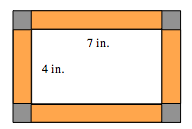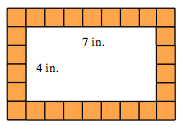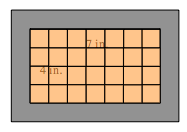### Home > MC1 > Chapter 3 > Lesson 3.4.2 > Problem3-108

3-108.
1. .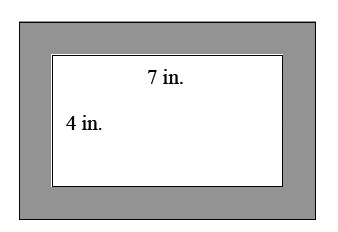Tina is going to put 1-inch square tiles on the picture frame shown at right. Homework Help ✎

1. If the width of the frame is one inch, how many 1-inch-square tiles will she need?

2. Would more 1-inch square tiles fit inside the frame or on the frame? Show how you know.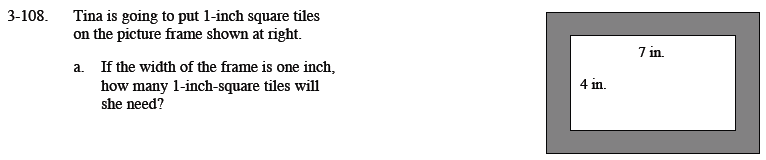To find the number of tiles, it may be useful to find the perimeter of the picture. Remember that the perimeter is the distance around something, so you will know how many tiles fit around the picture. If you use this strategy, do not forget to account for the corner tiles.

You can also draw square tiles with each side one inch long. This way you can count the tiles Tina needs.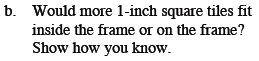The area of a shape is defined as the number of square tiles that fit in the interior of the shape. The area of a rectangle can be found by using this formula:
Area = (length)(width).

You can also find the number of tiles inside the frame (area) by drawing the tiles and then counting them.

Where do more tiles fit?